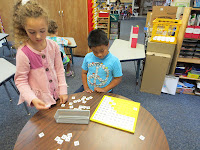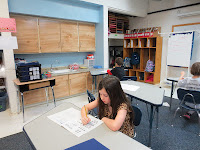### This Week in River Class

This week's Big Fish!

In first year math group this week the children have been learning about place value using manipulatives to represent tens and ones. Math students rolled the dice and were able to exchange their single cubes for a ten stick when they reached the number ten.

Students also took turns to write numbers on the board in order to practice number order and formation.

Second year math students continue to work through their Mastering Math workbooks.Students also solved word problems in their math notebooks. "There are 5 cows and 4 chickens. How many feet and tails altogether?"  Students drew pictures and wrote words to explain how they solved the problem.Math rotation includes ipads, (we used the Sumstacker app), completing the hundred board, and working on workbooks. We also have fun practicing subtraction and addition facts in pairs using flashcards.